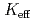In Monte Carlo criticality calculation (MCCC), each output quantity of interest is a series of tallies under autocorrelation. As a consequence from the functional central limit theorem, the stepwise interpolation of standardized tallies (SIST) converges in distribution to Brownian bridge (BB). Here, the standardization of tallies is a functional version of the statistic in the central limit theorem with the sample mean at each generation and the true mean replaced by the sample mean at the final generation. Fractional Brownian motion (FBM) is a family of stochastic processes and assumes a unique value of the box counting dimension (BCD) in fractal geometry. This work shows that the BCD of SIST is an effective diagnostic measure for the run length of MCCC. The sufficiency of the number of generations run can be judged by relating the BCD of FBM to that of BB. Numerical results are presented for power distribution tallies of a pressurized water reactor and the effective multiplication factor () tallies of the criticality problem by D. Mennerdahl.